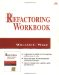# Chapter 7 . Conditional Logic

### Chapter 7. Conditional Logic

 Exercise 20. Null Object A. An empty string may not be the right choice for a default value in every context. B. It's possible that extracting a new class for Bin might give you the needed flexibility.

Exercise 21. Conditional Expression

A.

` if ((score <= 700) &&    ((income < 40000)  (income > 100000)       !authorized  (score <= 500)) &&    (income  <= 100000))   reject() else   accept(); `

B.

` boolean hasMidRangeIncome =   (income >= 40000) && (income <= 100000); boolean hasHighIncome = (income > 100000); boolean hasHighScore = (score > 700); boolean hasMidScore = (score > 500); if (! (hasHighScore       (hasMidRangeIncome && authorized && hasMidRangeScore)       hasHighIncome) )   reject(); else   accept(); `

C.

` if (score > 700)   accept(); else if ((income >= 40000) && (income <= 100000)       && authorized && (score > 500))   accept(); else if (income > 100000)   accept(); else   reject(); `

D.

` boolean acceptable(int income, int score, boolean authorized) {   if ((score > 700)  (income > 100000))     return true;   if ((income >= 40000) && (income <= 100000)       && authorized && (score > 500))     return true;   return false; } if (acceptable(income, score, authorized))   accept(); else   reject(); `

F.

HIGH INCOME

MEDIUM INCOME

LOW INCOME

AUTH=Y

AUTH=N

AUTH=Y

AUTH=N

AUTH=Y

AUTH=N

HIGH SCORE

Accept

Accept

Accept

Accept

Accept

Accept

MID SCORE

Accept

Accept

Accept

Reject

Reject

Reject

LOW SCORE

Accept

Accept

Reject

Reject

Reject

Reject

Or, alternatively:

HIGH INCOME

MEDIUM INCOME

LOW INCOME

HIGH SCORE

Accept

Accept

Accept

MID SCORE

Accept

Accept if authorized

Reject

LOW SCORE

Accept

Reject

Reject

 Exercise 22. Switch Statement A. If this were all there were to it, you might not bother eliminating the switch. But it would already be very natural to have print() and do() methods on operations to let us eliminate the type field. Exercise 23. Factory Method B. You can argue it either way, but it's starting to get kind of big for an enumeration of integers. C. What are some advantages to using dynamic class loading? The code is simpler (no conditional logic; a single place where class is created). The code has fewer direct dependencies (doesn't name the actual driver classes). The delivered code can be smaller (it's no longer necessary to deliver the debugging driver class ”nothing depends on it directly). New driver classes can be dynamically loaded without having to recompile the whole system. D. What are some disadvantages to this new arrangement? Performance is potentially a little worse . A simple text scan no longer reveals all the dependencies of the code. The configuration is a little trickier; an incorrect name or a bad CLASSPATH can leave the system unable to run.Refactoring Workbook
ISBN: 0321109295
EAN: 2147483647
Year: 2003
Pages: 146

Similar book on Amazon